# 【Python】深度讲解序列和字典,复习必备-太细啦

### 文章目录:

• 序列
• 序列的概念
• 标准类型操作符
• 序列类型操作符
• 序列的切片操作
• 翻转字符串
• 序列内建函数
• len函数
• max函数 -O(N)
• min函数 -O(N)
• sorted函数
• sum函数
• enumerate函数
• zip函数
• 字符串
• 三种引号的区别
• 理解字符串 "不可变"
• 字符串的标准类型操作符
• 只适用于字符串的操作符
• 原始字符串(raw strings)
• repr函数和反引号操作符
• string 模块常用函数
• 注意点:记得字符串是不可变对象, 只能生成新字符串
• join函数
• **split函数**
• **startswith函数 和 endswith函数**
• strip函数
• ljust rjust center函数
• find函数
• **replace函数**
• **isalpha函数 和 isdigit函数**
• **lower和upper函数**
• 关于结束符
• 列表 []
• 使用切片操作符访问列表元素
• 列表常用操作
• append: 追加元素
• 删除指定下标元素 del
• 按值删除元素 remove
• 列表比较操作
• in/not in: 判断一个元素在不在列表中
• 连接操作符(+): 连接两个列表
• extend: 列表连接
• 重复操作符(*):
• insert:任意位置插入
• reverse:原地翻转列表
• sort:原地排序
• count:统计元素出现次数
• index:相当于查找,返回下标
• pop:删除列表一个元素
• 以上函数注意点:
• 基于列表的堆栈
• 基于列表的队列
• 列表的深拷贝/浅拷贝(选学)
• 总结:
• 如何进行深拷贝:
• 元组 ()
• 元组常用操作
• 默认集合类型
• 理解元组的 "不可变"
• 元组不可以替代的原因
• 字典{}
• 创建字典
• 访问字典中的元素
• 修改字典元素
• 删除字典元素
• 常用内置函数
• in / not in:
• len函数
• hash函数
• keys
• values
• items
• 集合(set)
• 集合基本操作
• 取交集& 并集| 差集 – 对称差集^
• 数据去重 使用->set
• 本文章主要讲解内容：

理解Python的序列的基本概念.
掌握字符串/列表/元组/字典的基本使用方法.
理解列表和元组的区别和各自的应用场景.
理解Python中的深拷贝和浅拷贝.
理解字典 “键值对” 这样的概念

## 序列

### 序列的概念

包含若干个元素, 元素有序排列, 可以通过下标访问到一个或者多个元素. 这样的对象, Python中统一称为序列(Sequence).

Python中的以下对象都属于序列

• 字符串
• 列表
• 元组
• 同是序列, 他们的使用方式有很多相通之处

注意:序列里面的元素的顺序很重要,因为比较是按顺序比

``````a = [1,2,3]
b = [3,2,1]
print(a ==b)    #False
``````

### 标准类型操作符

下列标准类型操作符, 大多数情况下都是适用于序列对象的(少部分特例是, 序列中保存的元素不支持标准类型操作符).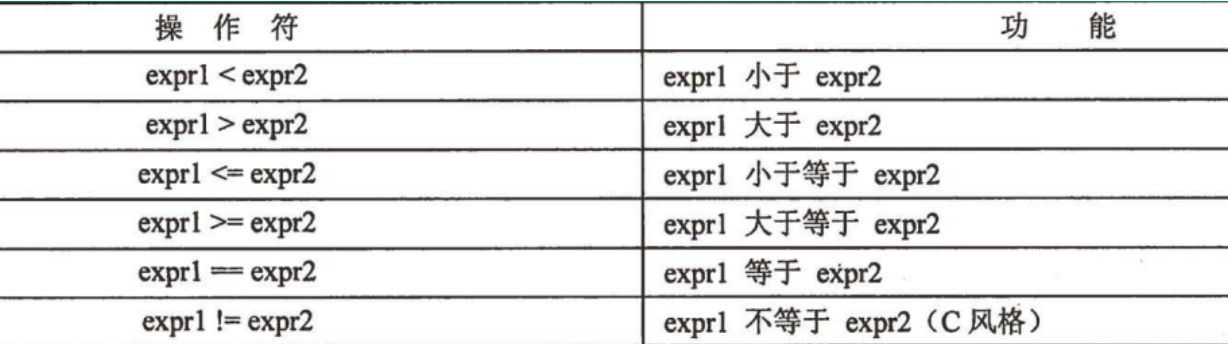### 序列类型操作符

• in/not in: 判定一个元素是否存在于序列中, 返回布尔值.
• ``````a = [1,2,3,4]
print(3 in a)	#True
print(3 not in a)	#False
``````
• 连接操作符(+): 把两个相同类型的序列进行连接.
• ``````a = [1,2,3,4]
b = [5,6]
print(a+b)	#返回一个新列表,包含了a和b的所有元素[1, 2, 3, 4, 5, 6]
``````
• 连接操作符往往并不高效(新创建对象, 把原有对象拷贝进去). 对于列表, 推荐使用extend来完成这样的操作; 对于字符串, 推荐使用join这样的方法.
• ``````a = [1,2,3,4]
b = [5,6]
a.extend(b)		#相当于把b的元素都插入到a的后面
print(a)	#[1, 2, 3, 4, 5, 6]
``````
• 重复操作符(*): 让一个序列重复N次.
• ``````a  =[1,2,3]
print(a*3)	#[1, 2, 3, 1, 2, 3, 1, 2, 3]
``````

### 序列的切片操作

• 切片操作符([ ], [A:B], [A:B:C]): 通过下标访问其中的某一个元素, 或者某个子序列
•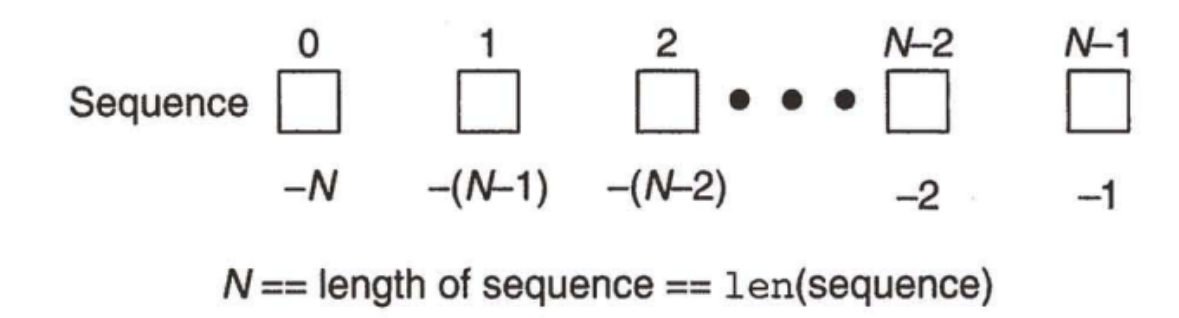• 正数的索引以序列的起始位置作为起点, 负数的索引以序列的结束位置做为起点.
• 试图访问一个越界的索引, 会引发异常(可以简单理解成程序执行出错)
• ``````a  =[1,2,3]
print(a)
#执行结果：
IndexError: list index out of range
``````

关于切片:左闭右开区间

方式1：[:] 左右两个端点都不写值,截取的是整个序列的元素,从头到尾

``````a  =[1,2,3,4,5]
print(a[:])#[1, 2, 3, 4, 5]
``````

方式2：[A:B]

元素下标取值范围: [A,B)

``````a  =[1,2,3,4,5]
print(a[1:3])		#[2,3]		 截取下标[1,3)的元素
print(a[1:-1])		#[2,3,4]	 截取下标[1,-1)的元素
print(a[:3])		#[1,2,3]	 截取下标[0,3)的元素
print(a[1:])		#[2,3,4,5]	 截取下标[1,-1)的元素
``````

如果左边端点不写,默认从0开始, 右边端点不写,默认截取到最后一个位置(即:-1位置)

方式3:[A:B:C] 第三个参数表示步长,即每隔多少个元素截取一个

• 扩展切片操作[::] 除了可以表示子序列的起始和结束位置, 还可以表示 “步长”
• 例子:

``````a = [1,2,3,4,5]
print(a[::2])   #每两个元素截取一个
#执行结果:
[1,3,5]
``````

#### 翻转字符串

字符串翻转, 这是一个非常基础, 也是笔试面试中会经常出现的一个题目. 我们学过C/C++, 有三种方法来解决这个问题.

方法1:首尾指针

``````char str[] = "abcdefg";
char* beg = str;
char* end = str + strlen(str);
while (beg < end) {
swap(*beg++, *--end);
}
``````

方法2:栈

``````char str[] = "abcdefg";
Stack stack;
char* p = str;
while(p) {
stack.push(*p++);
}
int index = 0;
while(!stack.empty()){
str[index++] = stack.top();
stack.pop();
}
``````

方法3:使用reverse + 迭代器翻转

``````#include <algorithm>
char str[] = "abcdefg";
std::reverse(str, str + strlen(str));	//指针就是天然的迭代器
``````

python的做法:

``````a = "abcdefg"
print(a[::-1])
``````

这个代码的含义:

`a[::-1]` -1表示往前走,从后往前拿元素

``````a = [1,2,3,4,5,6]
print(a[::-1])   	#[6,5,4,3,2,1]
#含义
从-1位置往前走,先走到下标为-1位置，然后从6开始往前走
``````

对于切片语法来说, 下标越界也没关系. 因为取的是前闭后开区间，区间里的元素, 能取到多少就取到
多少.

### 序列内建函数

##### len函数

len: 返回序列的长度.

``````a = [2,3,4,5]
print(len(a))	#4
b = "hello"
print(len(b))	#5
``````

##### max函数 -O(N)

max: 返回序列中的最大值

``````a = [2,3,4,5]
print(max(a))	#5
b = "helloz"
print(max(b))	#z
``````

##### min函数 -O(N)

min: 返回序列中的最小值

``````a = [2,3,4,5]
print(min(a))	#2
b = "helloz"
print(min(b))	#e
``````

##### sorted函数

sorted: 排序. 这是一个非常有用的函数. 返回一个有序的序列(输入参数的副本).

``````a = ['abc','acb','a','b']
print(sorted(a))	#['a', 'abc', 'acb', 'b']
a = [5,3,3,1,5]
print(sorted(a))	#[1, 3, 3, 5, 5]
``````

sorted可以支持自定制排序规则

##### sum函数

sum: 序列中的元素求和(要求序列中的元素都是数字)

``````a = [1,2,3,4,5]
print(sum(a))	#15
a= [1,'a']
print(sum(a))	#报错  unsupported operand type(s) for +: 'int' and 'str'
``````

##### enumerate函数

enumerate: 同时枚举出序列的下标和值 可以避免很多丑陋的代码.

例如:找出元素在列表中的下标

``````a = [1,2,3,4,5]
def Find(input_list,x):
for i in range(0,len(input_list)):
if input_list[i] == x:
return  i
else:   #此处的else和for搭配
return None

print(Find(a,2))	#1  下标为1
``````

这里用for循环写的就不够优雅,使用enumerate函数就可以写的很优雅

``````a = [1,2,3,4,5]
def Find(input_list,x):
for i ,item in enumerate(input_list):
if item == x:
return  i
else:   #此处的else和for搭配
return None

print(Find(a,2))	#1 下标为1
``````

##### zip函数

zip: 这个函数的本意是 “拉链”,

``````x = [1,2,3]
y = [4,5,6]
z = [7,8,9,10]	#多余的10不要，3行3列

print(zip(x,y,z))   #直接打印是对象的id	<zip object at 0x000001581CFE7748>
#把执行结果强转为list,列表
print(list(zip(x,y,z)))	#[(1, 4, 7), (2, 5, 8), (3, 6, 9)]

#直观打印
for i in zip(x,y,z):
print(i)
#执行结果：
(1, 4, 7)
(2, 5, 8)
(3, 6, 9)
``````

zip可以理解为行列互换

zip的一个比较常见的用法, 就是构造字典

``````key = ('name','id','score')
value =('Mango','2022','99')
d = dict(zip(key,value))	#执行结果转为一个字典
print(d)	#  {'name': 'Mango', 'id': '2022', 'score': '99'}
``````

## 字符串

### 三种引号的区别

• Python中单引号(')字符串和双引号(")字符串是等价的. 而不像有些编程语言(比如Linux Shell), 转义字符只在双引号中有效.
• Python中使用 “原始字符串” 来完成这样的功能
• 三引号(‘’'/“”")相比于前两种的区别是, 可以跨多行.
• 三引号还可以作为文档字符串

• ### 理解字符串 “不可变”

• 字符串实际上是不可变的对象. 你不能修改这个字符串, 只能创建一个新的字符串.
• ``````a = 'abcd'
a ='z'	#TypeError: 'str' object does not support item assignment

a = 'z'+a[1:]
print(a)	#zbcd
``````

### 字符串的标准类型操作符

• ==, !=, <, <=, >, >= 这些操作符的行为前面已经提到过.

• 需要记得字符串比较大小是按照字典序.

• ``````a = 'abc'
b = 'ab'
print(a != b)	#True
print(a < b)	#False	按照字典序比较
``````

• in/not in的规则和序列的规则一致.
• ``````a = 'abc'
print('a' in a)	#True
print('z' in a)	#False
``````
• 切片操作和序列规则一致
• ``````a = 'abcd'
print(a[1:2])   #b
print(a[:2])    #ab
print(a[1:])    #bcd
print(a[:])     #abcd
print(a[::2])   #ac
``````

### 只适用于字符串的操作符

• %: 格式化替换.
• ``````x = 1
print('x = %d' %x)	# x = 1
``````

``````x = 10
y = 20
a = 'x = %d y = %d' %x #缺少参数：报错 TypeError: not enough arguments for format string

#正解：
x = 10
y = 20
a = 'x = %d y = %d' %(x,y)
``````

推荐写法:加前缀f

``````x = 10
y = 20
a = f'x = {x},y={y}'
print(a)	#x = 10,y=20
``````

支持以下这些格式化字符串：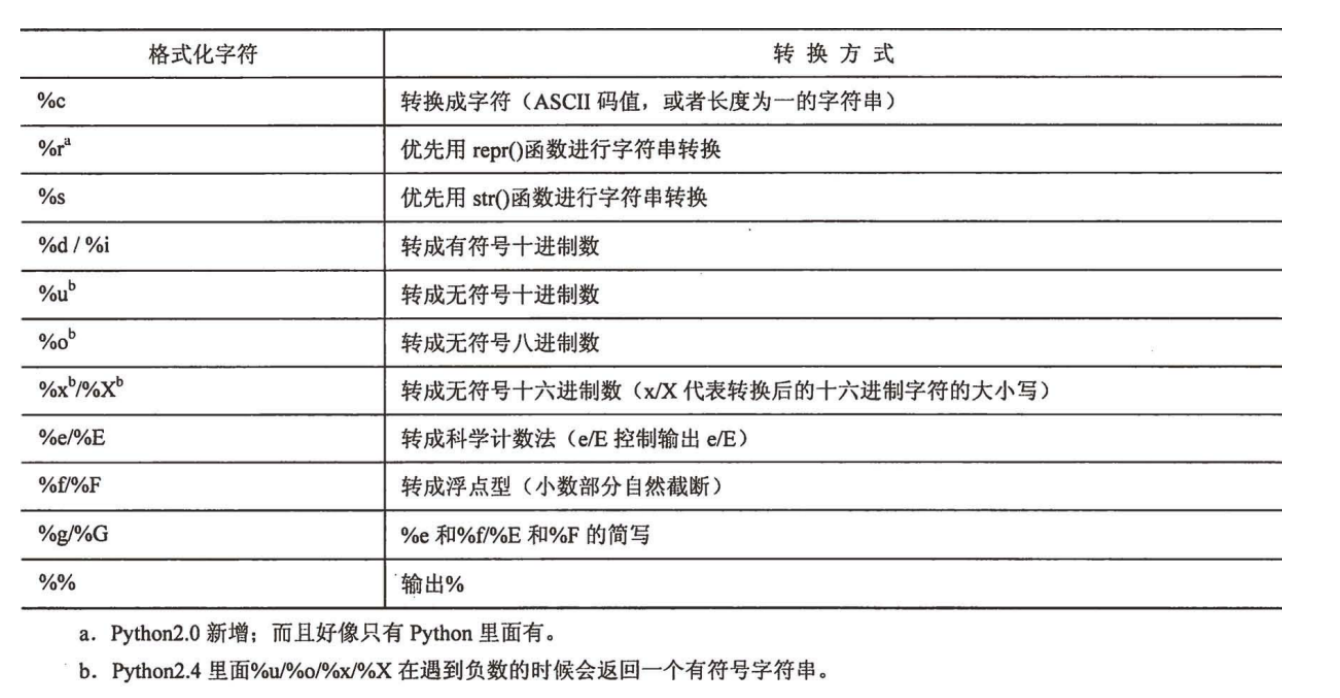### 原始字符串(raw strings)

有的时候, 我们需要有 \n 这样的字符作为转义字符**. 但是有些时候我们又不希望进行转义, 只需要原始的**
\n 作为字符串的一部分.

原始字符串中, 转义字符不生效

例子:QQ发消息时, 有一个 “表情快捷键” 的功能. 这个功能就相当于 “转义字符”.

当开启了这个功能之后, 在输入框中输入 /se 就会被替换成一个表情. 比如我给同事发一个目录 /search/odin (这本来是表示linux上的一个目录)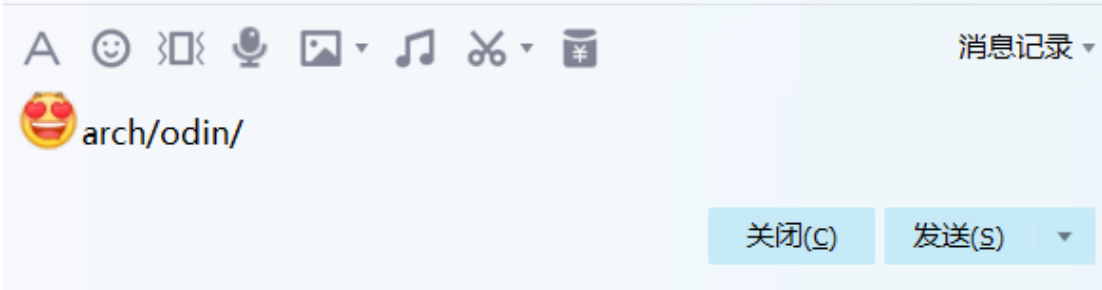这种情况下, 我们需要关闭 “表情快捷键” 功能. 对于Python来说, 我们就可以使用原始字符串来解决这个问题.

• 在字符串字面值前加上 r或者R 前缀, 表示原始字符串
• ``````print(r'hello \n world')	#hello \n world
``````

### repr函数和反引号操作符

• 用str函数可以将一些对象转换成字符串. repr也有类似的效果.
• ``````a = 1
print(type(repr(a)))    #<class 'str'> 字符串类型

print(str('hello'))		# hello
print(repr('hello'))	# 'hello'
``````

总结一下, str转换出的字符串是给人看的. 而repr转换出的字符串, 是给Python解释器看的.

• 意思是说, repr得出的结果, 其实是一个Python语句, 可以直接放到解释器里执行~

• 反引号, 和repr函数等价
• ### string 模块常用函数

• Python标准库提供了string模块, 包含了很多非常方便实用的函数
• #### 注意点:记得字符串是不可变对象, 只能生成新字符串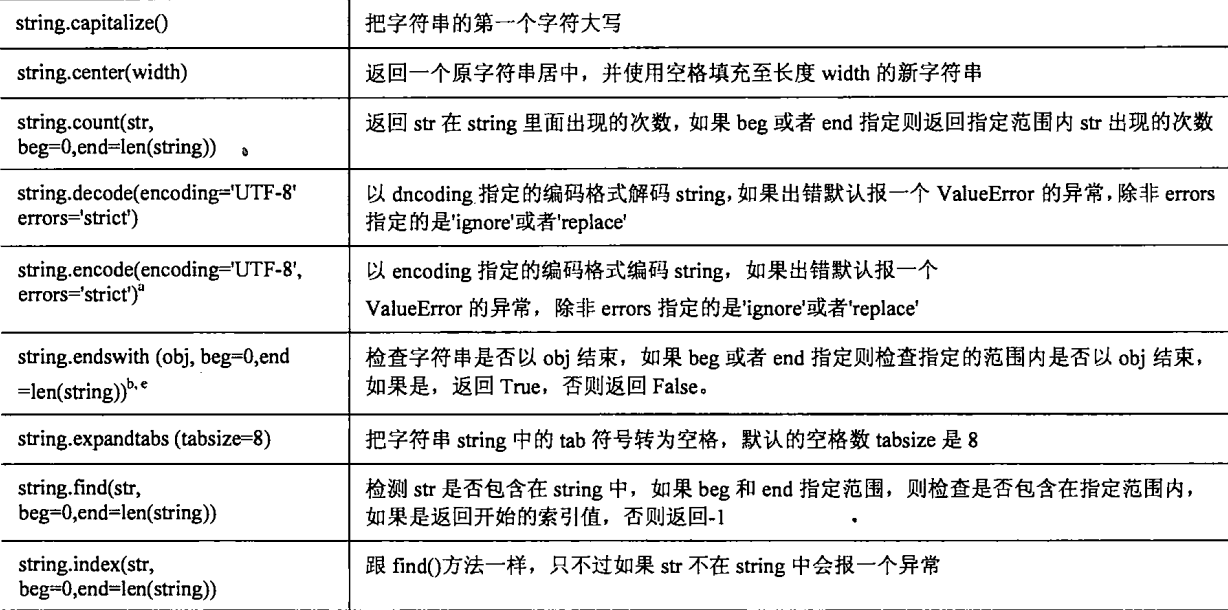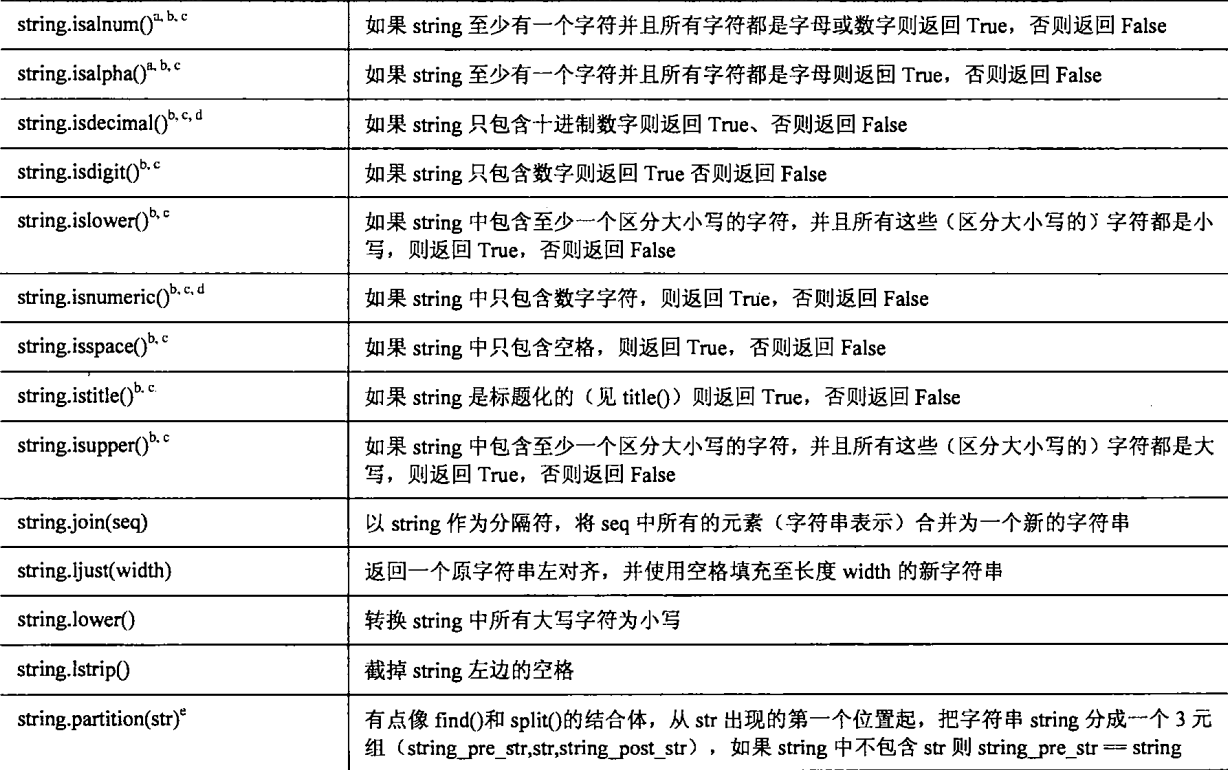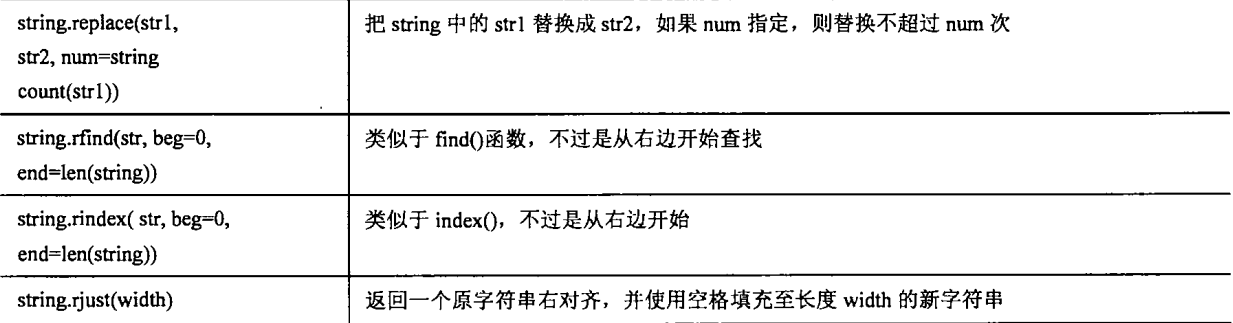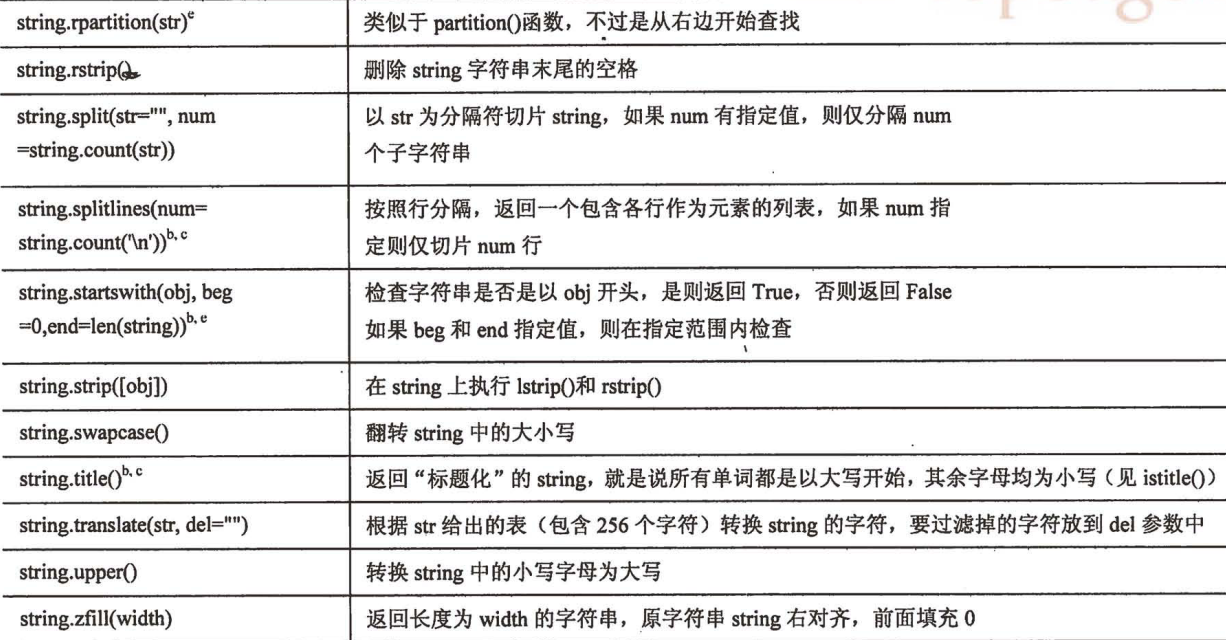##### join函数

将序列中的字符串合并成一个字符串. join函数

``````a = ['aa','bb','cc']
b = ' '.join(a)
print(b)	#aa bb cc
``````

##### split函数

按空格将字符串分割成列表 split函数

``````a = 'aa bb cc'
b = a.split(' ')
print(b)	#['aa', 'bb', 'cc']
``````

通常和join函数一起使用

``````a = 'aaa,bbb,ccc'
b = a.split(',') #以,分割成列表
print(b)
print(';'.join(b)) #分号连接
print('hello'.join(b))

#执行结果：
['aaa', 'bbb', 'ccc']
aaa;bbb;ccc
aaahellobbbhelloccc
``````

##### startswith函数 和 endswith函数

判定字符串开头结尾 startswith函数 和 endswith函数

``````a = 'hello world'
print(a.startswith('h'))	#True
print(a.startswith('hee'))	#False
print(a.endswith('d'))		#True
``````

##### strip函数

去除字符串开头结尾的空格/制表符 strip函数

空白字符：空格,换行,tab

``````a = '       hello world             '
print(a.strip())	#hello world
``````

去掉左侧的空白字符：lstrip

去掉右侧的空白字符： rstrip

``````a ='    hello   \n'
print(f'[{a.lstrip()}]') 	#为了方便看,加上[]
print(f'[{a.rstrip()}]')
print(f'[{a.strip()}]')
#执行结果：
[hello
]
[    hello]
[hello]
``````

##### ljust rjust center函数

左对齐/右对齐/中间对齐 ljust rjust center函数

``````a = '    hello world'
print(a.ljust(30))
print(a.rjust(30))
print(a.center(30))
#执行结果:
hello world
hello world
hello world
``````
##### find函数

查找子串 find函数

``````a = '    hello world'
print(a.find('hello'))	#4
a = 'hello hello '
print(a.find('h'))	#0
``````

返回第一次出现的下标

`in`差不多,in返回的是布尔值

``````a = '    hello world'
print(a.find('hello'))	#4
print('hello' in a)	#True
``````

##### replace函数

替换子串(记得字符串是不可变对象, 只能生成新字符串). replace函数

``````a=  'hello world'
print(a.replace('world','python'))	#hello python
print(a)	#hello world	字符串是不可变对象, 只能生成新字符串
``````

##### isalpha函数 和 isdigit函数

判定字符串是字母/数字 isalpha函数 和 isdigit函数

``````a = 'hello 1'
print(a.isalpha())	#False
a = '1234'
print(a.isdigit())	#True
``````

##### lower和upper函数

转换大小写 lower和upper函数

``````a = 'Hello world'
print(a.lower())	#hello world
print(a.upper())	#HELLO WORLD
``````

### 关于结束符

学过C语言的同学, 可能会问, Python的字符串是否需要 ‘\0’ 之类的结束符来做结尾?

• Python中并没有那个讨厌的 ‘\0’. 准确的说, 对于C语言来说, 语言本身的缺陷并不支持 “字符串类型”, 才被迫使用字符数组来凑合. 但是Python完全没有这个包袱.

• ## 列表 []

字符串只能由字符组成, 而且不可变; 但是列表中可以包含任意类型的对象, 使用更加灵活.

### 使用切片操作符访问列表元素

• 列表的切片操作和字符串完全一致.
• 但是列表还可以使用切片操作修改元素.
• ``````a = [1,2,3,4]
a = 100
print(a)	#[100,2,3,4]
``````

### 列表常用操作

#### append: 追加元素

把append的东西当成一个元素

``````a = [1,2,3]
a.append('a')
print(a)	#[1,2,3,'a']
``````

注意:使用append:是把元素当成整体插入

``````a = [1,2]
a.append([3,4])	#插入的是个整体
print(a)	#[1,2,[3,4]]		二维列表
print(len(a))	#3
``````

#### 删除指定下标元素 del

``````a = [1,2,3]
del(a)
print(a)	#[2,3]
``````

#### 按值删除元素 remove

``````a = [1,2,3]
a.remove(1)
print(a)    #[2, 3]
#如果元素不存在,就会报错
a.remove(4) #报错 ValueError: list.remove(x): x not in list
``````

#### 列表比较操作

== != 为判定所有元素都相等, 则认为列表相等;

< <= > >= 则是两个列表从第一个元素开始 依次比较, 直到某一方胜出. 按顺序比较

``````a = ['abc',12]
b = ['acb',123]
c = ['abc',11]
print(a<c)	#False
print(b<c)	#False
print(b >c and a > c)	#True

a = [1,2,3]
b = [3,2,1]
print(a <b)    #True 按顺序比

a = [1,2,3]
b = ['a','b']
print(a<b) 	#报错：TypeError: '<' not supported between instances of 'int' and 'str'
``````

#### in/not in: 判断一个元素在不在列表中

时间复杂度O(N)

``````a = [1,2,3,'a']
print('a' in a)	#True
print(4 in a)	#False
``````

#### 连接操作符(+): 连接两个列表

a和b都不变，生成新对象

``````a = [1,'a']
b = [2,'b']
print(a+b)	#[1, 'a', 2, 'b']
``````

#### extend: 列表连接

如果使用append:是把元素当成整体插入

``````a = [1,2]
a.append([3,4])	#插入的是个整体
print(a)	#[1,2,[3,4]]
print(len(a))	#3
``````

使用extend

可以理解成 += (列表不支持 += 运算符)

``````a = [1,2]
a.extend([3,4])
print(a)	#[1,2,3,4]
print(len(a))	#4
``````

#### 重复操作符(*):

``````a = [1,2]
print(a*3)	#[1, 2, 1, 2, 1, 2]
``````

#### insert:任意位置插入

如果下标很大,插入到最后面

insert没有返回值:

``````a =[1,2,3]
print(a.insert(0,0)) #None
``````

例子:

``````a=[1,2,3]
a.insert(0,0)	#在0下标位置插入0
a.insert(100,100)	#在下标100位置插入100
print(a)	#[0, 1, 2, 3, 100]
``````

#### reverse:原地翻转列表

``````a = [1,2,3,'ac']
a.reverse()
print(a)	#['ac', 3, 2, 1]
``````

也可以通过切片翻转 `a[::-1]`和reverse翻转的区别：

切片翻转不修改原来对象,生成新对象, reverse翻转修改原来对象

#### sort:原地排序

这个是列表内置的成员函数,和sorted不同

例如, 排序. 我们前面讲序列的时候, 有一个内建函数sorted, sorted生成了一个新的有序的序列. 列表自身还有一个成员函数sort, 是基于自己本身做修改, 并不生成新对象.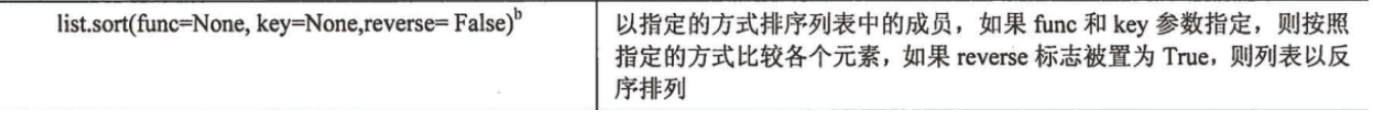``````a = [4,3,2,1]
sorted(a)#内建函数sorted
print(a)#[4, 3, 2, 1] a不改变
a.sort()#成员函数sort
print(a)#[1, 2, 3, 4]
a.sort(reverse=True)    #翻转
print(a)    #[4,3,2,1]
``````

关于sort方法, 默认使用归并排序的衍生算法. 时间复杂度是 O(N*log(N))

#### count:统计元素出现次数

``````a = [4,3,2,1,2,4,2]
print(a.count(2))	# 3
print(a.count(5))	# 0
``````

#### index:相当于查找,返回下标

``````a = [4,3,2,1]
print(a.index(4))	#0
print(a.index(5))	#报错 ValueError: 5 is not in list
``````

#### pop:删除列表一个元素

下标删除,默认值是-1,删最后一个元素

如果列表为空还弹出,就会报错

``````a =
print(a)	#
a.pop()
print(a)	#[]
a.pop()	#列表为空还弹出,报错：IndexError: pop from empty list
``````

还可以给下标删除:如果下标越界会报错

``````a = [1,2,3,4]
a.pop(3)	#删除下标为3的元素
print(a)	#[1,2,3]
a.pop(6)	#报错 IndexError: pop index out of range
``````

##### 以上函数注意点:

列表自身是可变对象, 因此有些方法是修改自身的, 这种情况下的方法并没有返回值,

这和字符串操作必须要生成一个新对象, 并不相同.

### 基于列表的堆栈

我们回顾一下数据结构中的堆栈, 这是一种后进先出的数据结构. 可以很容易使用列表进行模拟

``````a = [] #空列表
a.append(1) #push操作  在栈顶插入元素
print(a[-1])  #top操作    得到栈顶元素
a.pop() #pop操作  弹出栈顶元素
``````

### 基于列表的队列

我们回顾一下数据结构中的队列, 这是一种先进先出的数据结构. 也可以很容易使用列表模拟

``````a = [] #空列表
a.append(1) #push操作  在队尾插入元素
print(a)  #top操作    得到队头元素
a.pop(0) #pop操作  弹出队头元素
``````

### 列表的深拷贝/浅拷贝(选学)

``````#定义一个列表对象a.
a = [100, [1, 2]]
#基于a, 用三种方式创建了b, c, d
b = a
c = list(a)
d = a[:]
print(id(a), id(b), id(c), id(d))	#a和b同 ,c,d不同 b和a实际上是同一个对象. 而c和d都已经是新的列表对象了.

a = 1
print(a, b, c, d)	#a和b受影响  c和d不受影响

a = 1000
print(a, b, c, d)	#四个都受影响
print(id(a), id(c), id(d))	#三者相同

# 执行结果
140541879626136 140541879626136 140541879655672 140541879655744
[1, [1, 2]] [1, [1, 2]] [100, [1, 2]] [100, [1, 2]]
[1, [1000, 2]] [1, [1000, 2]] [100, [1000, 2]] [100, [1000, 2]]
139973004362816 139973004362816 139973004362816
``````

结果稍微有点复杂, 我们一点一点分析

• 定义一个列表对象a.
• 基于a, 用三种方式创建了b, c, d
• 首先可以看到, b和a实际上是同一个对象. 而c和d都已经是新的列表对象了.
• 修改 a 可以看到, a和b的值都收到了影响. 但是c和d不受影响, 因为a和b是同一个对象 c和d是新对象
• 再修改 a=1000 这时候惊奇的发现, c和d的内容也收到影响了!!!
• 这时候我们猜测, a, c, d的下标为1的列表元素, 其实都是同一个对象. 打印出id, 证明了我们的猜测.
•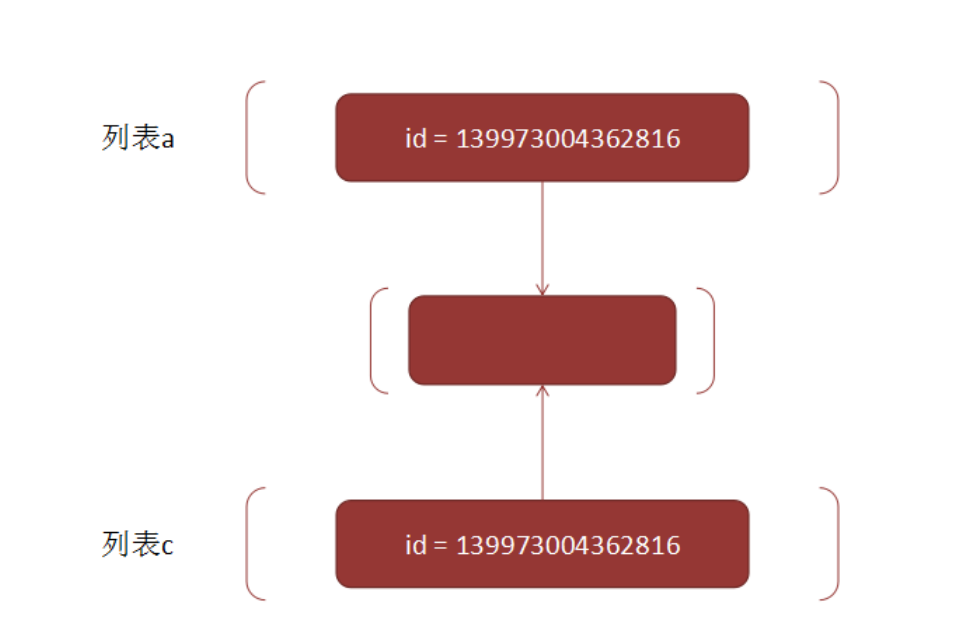#### 总结:

• 列表赋值(=)没有创建新对象, 但是list工厂函数和切片操作都创建了新的列表对象.
• 列表的赋值(=), 切片操作([:]), list工厂函数, 对象内部元素 都是在进行浅拷贝.
• 浅拷贝是指, 虽然我重新创建了一个新对象, 但是新对象里面包含的子对象, 和原来的子对象是同一个对象.
• 深拷贝就是指, 重新创建了一个新对象, 同时这个新对象的子对象, 也都是重新创建了一份新的深
拷贝对象.
• 深拷贝通过 `copy.deepcopy `来完成

• #### 如何进行深拷贝:

注意：深拷贝 只能针对可变对象使用

进行深拷贝： 需要导入copy模块

``````import copy
a = [100, [1, 2]]
b = copy.deepcopy(a)
print(id(a), id(b) )
# 执行结果
139818205071552 139818205117400
``````

这次我们看到, a 和 b 不再是一个对象了.

• 不光是列表, 其他容器, 例如元组, 字典, 也存在类似的深浅拷贝问题. 原理和列表相同
• 需要注意的是, 并不一定调用了deepcopy就一定会执行深拷贝, Python解释器自己也会根据具体的情况,比如某些不适合深拷贝的情况, 不会触发深拷贝.

• ``````import copy
a = (100, 'aaa')
b = copy.deepcopy(a)
print(id(a), id(a), id(b), id(b))
# 执行结果
6783664 140700444542616 6783664 140700444542616
``````

想想这是为啥?

进行深拷贝的目的:防止其中一份数据改了,会影响其它数据的结果

深拷贝就是为了改一个另外一个不变,但如果是不可变对象,都不允许修改,需求都不存在,

因为元组的内容本身就不能修改,没必要进行深拷贝!

## 元组 ()

元组的很多操作都和列表一致. 唯一的区别是元组的内容是只读的.

### 元组常用操作

切片操作: 和列表相同

比较运算符, 规则和列表相同

连接操作(+), 重复操作(*), 判定元素存在(in/not in)都和列表相同.

**由于元组不可变, 所以并不支持append, extend, sort,[],等修改自身的操作.**当然了,也不支持深拷贝

### 默认集合类型

• 所有的多个对象, 按逗号分隔的, 其实都是元组
• 回忆我们之前那个牛逼闪闪的交换两个元素的值的代码.

``````x, y = y, x
``````

其实, x, y 就是一个元组. 通过 y, x 这个元组创建了一个新的元组

``````x = 1
y = 2
x,y = y,x
print(type((x,y)))  #<class 'tuple'>
``````

### 理解元组的 “不可变”

• 元组的 “不可变” 指的是元组的每个元素的id不可变. 就是说一个元组包含了几个对象, 然后不可以给这个元组再添加或者删除其中的某个对象, 也不可以将某个对象改成其他的对象.
• 如果元组中的某个元素, 是可变对象(比如列表或字典), 那么仍然是可以修改的.
• ``````a = ([1,2],[3,4])
a = 100
print(a)	#([100, 2], [3, 4])
``````

元组:用`，`逗号分开元素

``````a = (10)    #因为可能是(10+2)*2这种表达式,所以a是整数,不是元组
print(type(a))  #<class 'int'>
a = (10,2)
print(type(a))  #<class 'tuple'>
``````

注意：

此处用的是元组重复操作符(*)的概念.

``````a = (10+20,) *3	#此处没有逗号,a就是90
print(a)    #(30, 30, 30)
print(type(a))  #<class 'tuple'>  元组
``````

“不可变” 真的有必要嘛? 假如我们只是用列表, 能否应付所有的应用场景呢?

答案是否定的. 既然Python的设计者提供了元组这个对象, 那么一定有一些情况下, 只有元组能胜任, 但是列表无法胜任.

#### 元组不可以替代的原因

原因1:函数传参的时候使用元组可以避免函数内部把函数外部的内容修改掉

你有一个列表, 现在需要调用一个API进行一些处理. 但是你有不是特别确认这个API是否会把你的列表数据弄乱. 那么这时候传一个元组就安全很多.

``````a = [1,2,3]
def func(input_list):
input_list = 100

func(a)
print(a)	#[100,2,3] 列表可以修改

#如果用的是元组:
a = (1,2,3)
def func(input_list):
input_list = 100

func(a)	#报错：TypeError: 'tuple' object does not support item assignment
print(a)
``````

原因2：元组是可哈希的,元组就可以作为字典的key,而列表是可变的,列表不可以作为字典的key

我们马上要讲的字典, 是一个键值对结构. 要求字典的键必须是 “可hash对象” (字典本质上也是一个hash表). 而一个可hash对象的前提就是不可变. 因此元组可以作为字典的键, 但是列表不行.

``````a = (1,2,3)
b = {
a:100
}
print(b) # {(1, 2, 3): 100}

#如果是列表:报错
a = [1,2,3]
b = {
a:100	#TypeError: unhashable type: 'list'
}
print(b)
``````

## 字典{}

Python的字典是一种映射类型数据. 里面的数据是 键值对 . Python的字典是基于hash表实现的.

• 键(key): 字典的键是唯一的(一个字典对象中不能有两个相同的键). 同时字典的键必须是可hash的(能够计算出一个固定的hash值)
• 值(value): 字典的值没啥限制. 数字, 字符串, 以及其他容器, 都可以作为字典的值

• 注意:字典的键和值的类型, 不需要相同

不同的键值用`，`逗号分割

``````a = {
10:20,
'key':'value',
'a':True
}
print(a)    #{10: 20, 'key': 'value', 'a': True}
``````

字典的key必须是可哈希的,不可变是可hash的必要条件

• 可以使用hash()函数判断是否可以哈希
• ``````print(hash((10,20)))    #元组可以哈希	3713074054217192181
print(hash([1,2]))  #列表不可以哈希	报错：TypeError: unhashable type: 'list'
``````

### 创建字典

• 使用 { } 的方法
• ``````a = {'name':"Mango",'age':20}
print(a)	#{'name': 'Mango', 'age': 20}
``````
• 使用工厂方法 dict

元组作为参数,元组的每个参数是个列表,列表里面有两个值: 一个是键 一个是值

• ``````a = dict((['name',"Mango"],['age',20]))
print(a)	#{'name': 'Mango', 'age': 20}
``````

• 使用字典的内建方法 fromkeys
• ``````a = {}.fromkeys(('name',"Ename"),"Mango")
print(a)	#{'name': 'Mango', 'Ename': 'Mango'}

a = {}.fromkeys(('name'),"Mango")
print(a)	#{'n': 'Mango', 'a': 'Mango', 'm': 'Mango', 'e': 'Mango'}
``````

注意:字典的键和值的类型,不需要相同.

### 访问字典中的元素

• 使用 [] 可以获取到元素的值.
• 注意:键是字符串,所以访问的是也要是字符串形式
• ``````a = {'name':"Mango",'age':20}
print(a['name'])	#Mango
print(a['age'])	#20
``````
• for循环遍历字典中的元素
• ``````a = {'name':"Mango",'age':20}
for item in a:  #item相当于每个键
print(item,a[item])   #打印键 和 值
#执行结果：
name Mango
age 20
``````

注意:字典中的键值对, 顺序是不确定的(回忆hash表的数据结构, 并不要求key有序).

### 修改字典元素

使用 [] 可以新增/修改字典元素. -> 如果key不存在, 就会新增; 如果已经存在, 就会修改.

``````a = {} #空字典
a['name'] ="Mango"
print(a)	#{'name': 'Mango'}
a['name'] = "Lemon"
print(a)	#{'name': 'Lemon'}
``````

### 删除字典元素

• 使用del来删除某一个键值对
• ``````a = {'name':"Mango",'age':20}
del a['name']
print(a)	#{'age': 20}
``````
• 使用clear方法, 清空整个字典中所有的键值对
• ``````a = {'name':"Mango",'age':20}
a.clear()
print(a)	#{} 空字典
``````
• 使用pop函数, 删除键值对, 同时获取到值
• ``````a = {'name':"Mango",'age':20}
b = a.pop('name')
print(a)	#  {'age': 20}
print(b)	#  Mango
``````

注意:字典也是可变对象. 但是键值对的key是不能修改的

### 常用内置函数

#### in / not in:

**判定一个key是否在字典中 ** (判断的是key,不是value)

``````a = {'name':"Mango",'age':20}
print('name' in a)		#True
print('Mango' in a)		#False
``````

#### len函数

len: 字典中键值对的数目

``````a = {'name':"Mango",'age':20}
print(len(a))	#2
``````

#### hash函数

hash: 判定一个对象是否可hash. 如果可hash, 则返回hash之后的hashcode. 否则会运行出错.

``````print(hash(())) #3527539  							  元组不可变,可以哈希
print(hash([])) #TypeError: unhashable type: 'list'   列表可变,不可哈希
``````

#### keys

返回一个列表, 包含字典的所有的key

``````a = {'name':"Mango",'age':20}
print(a.keys())	#dict_keys(['name', 'age'])
``````

#### values

返回一个列表, 包含字典的所有value

``````a = {'name':"Mango",'age':20}
print(a.values())	#dict_values(['Mango', 20])
``````

#### items

返回一个列表, 每一个元素都是一个元组, 包含了key和value

``````a = {'name':"Mango",'age':20}
print(a.items())	#dict_items([('name', 'Mango'), ('age', 20)])
``````

## 集合(set)

集合是基于字典实现的一个数据结构. 集合数据结构实现了很多数学中的交集, 并集, 差集等方法, 可以很方便的进行这些操作.

注意!! 熟练掌握集合操作, 对我们后续解笔试题面试题, 都会有很大帮助.

### 集合基本操作

集合和列表不同,集合的顺序没有影响

``````a = set([1,2,3])
b = set([3,2,1])
print(a == b)	#True
print(a,b)	#{1, 2, 3} {1, 2, 3}
``````

#### 取交集& 并集| 差集 – 对称差集^

``````a = set([1, 2, 3])
b = set([1, 2, 3, 4])
print(a & b) # 取交集
print(a | b) # 取并集
print(b - a) # 取差集
print(a ^ b) # 取对称差集(项在a中或者在b中, 但是不会同时存在于a和b中)
#执行结果
{1, 2, 3}
{1, 2, 3, 4}
{4}
{4}
``````

#### 数据去重 使用->set

``````a =set( [1, 1, 2, 2, 3, 3])
print(a)	#{1,2,3}

a = [1,2,1,11,1,1,2]
b = set(a)
print(a)	#[1, 2, 1, 11, 1, 1, 2]
print(b)	#{1, 2, 11}
``````

来源：芒果再努力